Quantitative MCQ - 24

# Quantitative MCQ - 24

Test Description

## 30 Questions MCQ Test Quantitative Aptitude for Competitive Examinations | Quantitative MCQ - 24

Quantitative MCQ - 24 for Quant 2022 is part of Quantitative Aptitude for Competitive Examinations preparation. The Quantitative MCQ - 24 questions and answers have been prepared according to the Quant exam syllabus.The Quantitative MCQ - 24 MCQs are made for Quant 2022 Exam. Find important definitions, questions, notes, meanings, examples, exercises, MCQs and online tests for Quantitative MCQ - 24 below.
Solutions of Quantitative MCQ - 24 questions in English are available as part of our Quantitative Aptitude for Competitive Examinations for Quant & Quantitative MCQ - 24 solutions in Hindi for Quantitative Aptitude for Competitive Examinations course. Download more important topics, notes, lectures and mock test series for Quant Exam by signing up for free. Attempt Quantitative MCQ - 24 | 30 questions in 30 minutes | Mock test for Quant preparation | Free important questions MCQ to study Quantitative Aptitude for Competitive Examinations for Quant Exam | Download free PDF with solutions
 1 Crore+ students have signed up on EduRev. Have you?
Quantitative MCQ - 24 - Question 1

### Directions (1-5): In the following questions, two equations numbered I and II are given. You have to solve both the equations and give answer\ I. √x-√6/√x=0 II. y³-6^((3⁄2) )=0

Detailed Solution for Quantitative MCQ - 24 - Question 1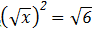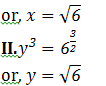Quantitative MCQ - 24 - Question 2

### Directions (1-5): In the following questions, two equations numbered I and II are given. You have to solve both the equations and give answer\  I. 3x-2y=10 II. 5x-6y=6

Detailed Solution for Quantitative MCQ - 24 - Question 2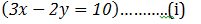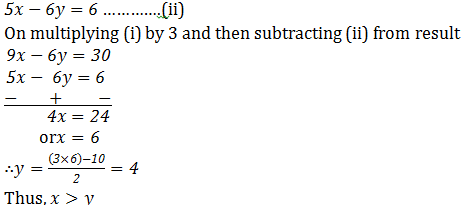Quantitative MCQ - 24 - Question 3

### Directions (1-5): In the following questions, two equations numbered I and II are given. You have to solve both the equations and give answer I. x²+x-12=0 II. y²-5y+6=0

Detailed Solution for Quantitative MCQ - 24 - Question 3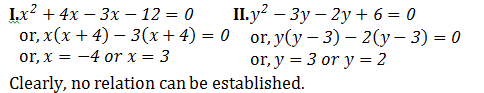Quantitative MCQ - 24 - Question 4

Directions (1-5): In the following questions, two equations numbered I and II are given. You have to solve both the equations and give answer\

I. x²+9x+18=0
II. y²-13y+40=0

Detailed Solution for Quantitative MCQ - 24 - Question 4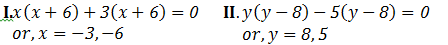Quantitative MCQ - 24 - Question 5

Directions (1-5): In the following questions, two equations numbered I and II are given. You have to solve both the equations and give answer

I. √(x+6)=√121-√36
II. y²+112=473

Detailed Solution for Quantitative MCQ - 24 - Question 5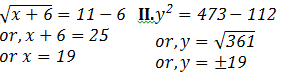Quantitative MCQ - 24 - Question 6

Directions (6-10) : What should come in place of the question mark (?) in the following number series.
325, 314, 292, 259, 215, ?

Detailed Solution for Quantitative MCQ - 24 - Question 6

The Pattern of the number series is :
325 – 1 × 11 = 314
314 – 2 × 11 = 292
292 – 3 × 11 = 259
259 – 4 × 11 = 215
215 – 5 × 11 = 160

Quantitative MCQ - 24 - Question 7

Directions (6-10) : What should come in place of the question mark (?) in the following number series.
45, 46, 70, 141,  ?, 1061.5

Detailed Solution for Quantitative MCQ - 24 - Question 7

Series is ×1+1,×1.5+1,×2+1,×2.5+1,……..

Quantitative MCQ - 24 - Question 8

Directions (6-10) : What should come in place of the question mark (?) in the following number series.
620,632, 608, 644, 596, ?

Detailed Solution for Quantitative MCQ - 24 - Question 8

Series is +(1×12),-(2×12),+(3×12),-(4×12),+(5×12),…….

Quantitative MCQ - 24 - Question 9

Directions (6-10) : What should come in place of the question mark (?) in the following number series.
15, 25, 40, 65, ?, 195

Detailed Solution for Quantitative MCQ - 24 - Question 9

Series is ×2-(1×5),×2-(2×5),×2-(3×5),×2-(4×5),……

Quantitative MCQ - 24 - Question 10

Directions (6-10) : What should come in place of the question mark (?) in the following number series.
120, 320, ?, 2070, 5195, 13007.5

Detailed Solution for Quantitative MCQ - 24 - Question 10

Series is ×2.5+20,×2.5+20,×2.5+20,…….

Quantitative MCQ - 24 - Question 11

A batsman has certain average of runs is 11 innings. In 12th innings he made 90 runs and thus the average is decreased by 5. His average after 12th inning is

Detailed Solution for Quantitative MCQ - 24 - Question 11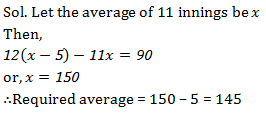Quantitative MCQ - 24 - Question 12

A seller labels the marked price of his goods 30% above the cost price but allows a discount of 15% on cash payment. What will be his percentage profit in cash deals?

Detailed Solution for Quantitative MCQ - 24 - Question 12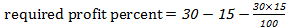= 10.5

Quantitative MCQ - 24 - Question 13

Pankaj bought a new house. 2% of the total price of the house will be given to broker as commission. 5% of the price of house is Rs. 2.5 lacs then what is the amount earned by the broker?

Detailed Solution for Quantitative MCQ - 24 - Question 13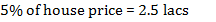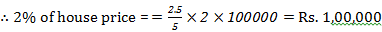Quantitative MCQ - 24 - Question 14

The difference between compound interest and simple interest on a certain sum at 10% per annum for 3 years is Rs. 124. What is the sum?

Detailed Solution for Quantitative MCQ - 24 - Question 14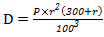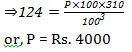Quantitative MCQ - 24 - Question 15

Direction (15-19): The following pie charts show the distribution of students of class 10th and 12th in seven different schools namely P, Q, R, S, T, U and V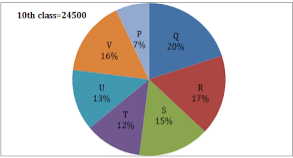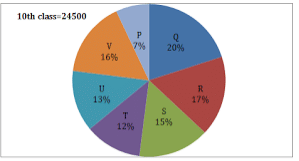Student studying in class 10th in school U are more than the students studying in class 12th of school R by what number?

Detailed Solution for Quantitative MCQ - 24 - Question 15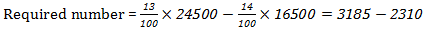= 875

Quantitative MCQ - 24 - Question 16

Direction (15-19): The following pie charts show the distribution of students of class 10th and 12th in seven different schools namely P, Q, R, S, T, U and VWhat is the difference between total number of students studying in class 10th in school R, S, T, & U together and students studying in class 12th in school P, Q, and V together?

Detailed Solution for Quantitative MCQ - 24 - Question 16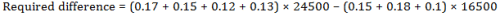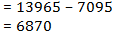Quantitative MCQ - 24 - Question 17

Direction (15-19): The following pie charts show the distribution of students of class 10th and 12th in seven different schools namely P, Q, R, S, T, U and V42% of students studying in class 10th in school Q failed in an examination. If the ratio of boys and girls in the failed group is 4 : 3, then find the number of girls who failed in the examination.

Detailed Solution for Quantitative MCQ - 24 - Question 17

Required number of girls who failed the examination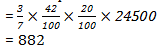Quantitative MCQ - 24 - Question 18

Direction (15-19): The following pie charts show the distribution of students of class 10th and 12th in seven different schools namely P, Q, R, S, T, U and VThe average number of students studying in class 12th of school S & T together are approximately what percent more than the number of students studying in class 10th of school P?

Detailed Solution for Quantitative MCQ - 24 - Question 18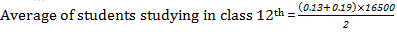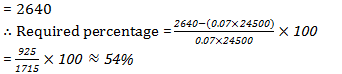Quantitative MCQ - 24 - Question 19

Direction (15-19): The following pie charts show the distribution of students of class 10th and 12th in seven different schools namely P, Q, R, S, T, U and VIn a fest, it was decided to select 30% of students studying in class 10th& 20% of students studying in class 12th from every school. What is the average number of students selected for the fest?

Detailed Solution for Quantitative MCQ - 24 - Question 19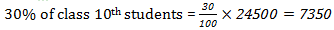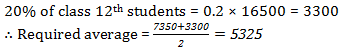Quantitative MCQ - 24 - Question 20

In a class of 240 students, each student got sweets that are 15% of the total number of students. How many sweets were there?

Detailed Solution for Quantitative MCQ - 24 - Question 20

No. of student = 240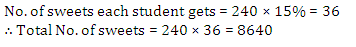Quantitative MCQ - 24 - Question 21

Seema purchased an item for Rs 9,600 and solid it for a loss of 5 percent. From that money, she purchased another item and sold it for a gain of 5 percent. What was her overall gain/loss?

Detailed Solution for Quantitative MCQ - 24 - Question 21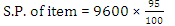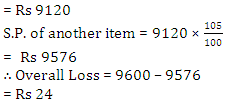Quantitative MCQ - 24 - Question 22

The compound interest (in Rupees) on Rs 5,600 for 3/2 years at 10% per annum, compounded annually is

Detailed Solution for Quantitative MCQ - 24 - Question 22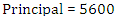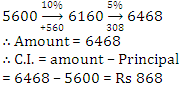Quantitative MCQ - 24 - Question 23

B is 3 years older than A and B is also 3 years younger than ‘C’. 3 years hence, the respective ratio between the ages of A and C will be 4: 5. What is the sum of the present ages of A, B and C?

Detailed Solution for Quantitative MCQ - 24 - Question 23

Let the present age of A = x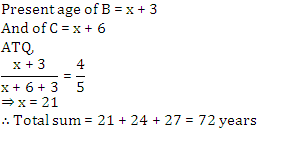Quantitative MCQ - 24 - Question 24

The average age of 80 boys in a class is 15 years. The average age of a group of 15 boys in the class is 16 years and the average age of another 25 boys in the class is 14 years. What is the average age of the remaining boys in the class?

Detailed Solution for Quantitative MCQ - 24 - Question 24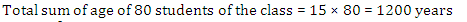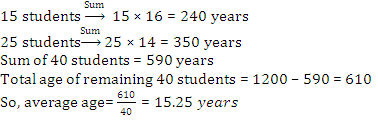Quantitative MCQ - 24 - Question 25

Directions (25-29): What should come in place of question mark (?) in the following number series?

2, 13, 67, ?, 817, 1639

Detailed Solution for Quantitative MCQ - 24 - Question 25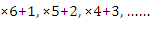Quantitative MCQ - 24 - Question 26

Directions (25-29): What should come in place of question mark (?) in the following number series?

1, 2, 6, 21, ?, 445, 2676

Detailed Solution for Quantitative MCQ - 24 - Question 26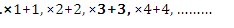Quantitative MCQ - 24 - Question 27

Directions (25-29): What should come in place of question mark (?) in the following number series?

8, 52, ?, 1287, 4504.5, 11261.25, 16891.875

Detailed Solution for Quantitative MCQ - 24 - Question 27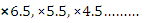Quantitative MCQ - 24 - Question 28

Directions (25-29): What should come in place of question mark (?) in the following number series?

250000, 62500, 12500, 3125, 625, ?, 31.25

Detailed Solution for Quantitative MCQ - 24 - Question 28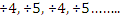Quantitative MCQ - 24 - Question 29

Directions (25-29): What should come in place of question mark (?) in the following number series?

588, 563, 540, 519, ?, 483, 468

Detailed Solution for Quantitative MCQ - 24 - Question 29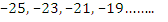Quantitative MCQ - 24 - Question 30

A train travelling at 48 km/hr crosses another train, having half its length and travelling in opposite direction at 42 km/hr in 12 seconds. It also passed a railway platform in 45 seconds. The length of the rail platform is

Detailed Solution for Quantitative MCQ - 24 - Question 30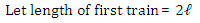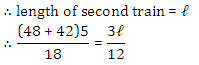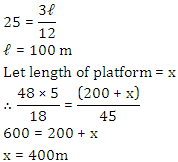## Quantitative Aptitude for Competitive Examinations

33 videos|34 docs|143 tests
 Use Code STAYHOME200 and get INR 200 additional OFF Use Coupon Code
Information about Quantitative MCQ - 24 Page
In this test you can find the Exam questions for Quantitative MCQ - 24 solved & explained in the simplest way possible. Besides giving Questions and answers for Quantitative MCQ - 24, EduRev gives you an ample number of Online tests for practice

## Quantitative Aptitude for Competitive Examinations

33 videos|34 docs|143 tests